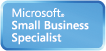Welcome Guest, you are in: Login

# MUST Creative Engineering Laboratory

### Technical Doc

Search the wiki
»

www.must.or.kr# SVM – Support Vector Machines

Modified on 2010/05/22 18:00 Categorized as Data Mining• Linear Support Vector Machine

Given a set of pointswith labelThe SVM finds a hyperplane defined by the pair (w,b)

(where w is the normal to the plane and b is the distance from the origin)

s.t.• What if the data is not linearly separable?

• Project the data to high dimensional space where it is linearly separable and then we can use linear SVM – (Using Kernels)Classification using SVM (w,b)In non linear case we can see this asKernel – Can be thought of as doing dot product in some high dimensional space

Example of Non-linear SVMSVM
• Relatively new concept
• Nice Generalization properties
• Hard to learn – learned in batch mode using quadratic programming techniques
• Using kernels can learn very complex functions